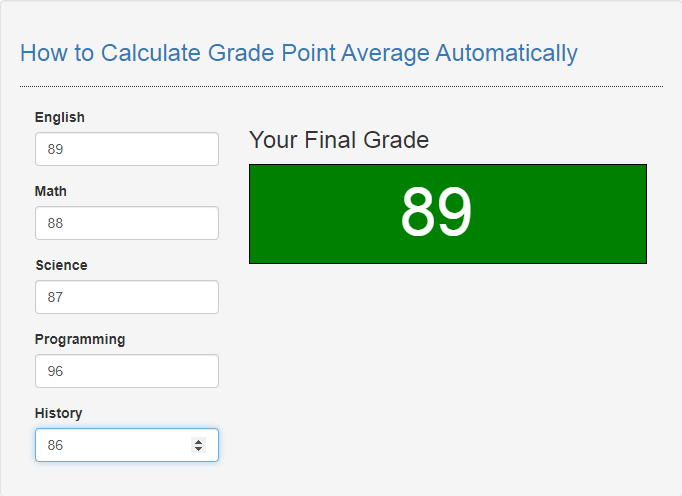# How to Calculate Grade Point Average Automatically in JavaScript

## Introduction

In this tutorial we will create a How to Calculate Grade Point Average Automatically in JavaScript. This tutorial purpose is to teach you how you can calculate the student gpa automatically. This will tackle all the basic function that will calculate the gpa. I will provide a sample program to show the actual coding of this tutorial.

This tutorial is simple and easy to understand just follow the instruction I provided and you can do it without a problem. This program can be use to any system or application that needed to compute the student gpa. I will give my best to provide you the easiest way of creating this program Calculate GPA automatically. So let's do the coding.

## Before we get started:

This is the link for the template that i used for the layout design https://getbootstrap.com/.

## Creating The Interface

This is where we will create a simple interface for our application. This code will only display the html forms to calculate the gpa. To create this simply copy and write it into your text editor, then save it as index.html.
`<!DOCTYPE html><html lang="en">	<head>		<meta charset="UTF-8" name="viewport" content="width=device-width, initial-scale=1"/>		<link rel="stylesheet" type="text/css" href="css/bootstrap.css"/>	</head><body>	<nav class="navabar navbar-default">		<div class="container-fluid">			<a class="navbar-brand" href="https://sourcecodester.com">Sourcecodester</a>		</div>	</nav>	<br />	<div class="col-md-3"></div>	<div class="col-md-6 well">		<h3 class="text-primary">How to Calculate Grade Point Average Automatically</h3>		<hr style="border-top:1px dotted;"/>		<div class="col-md-4">			<div class="form-group">				<label>English</label>				<input type="number" id="english" onkeyup="calculateAve();" class="form-control"/>			</div>			<div class="form-group">				<label>Math</label>				<input type="number" id="math" onkeyup="calculateAve();" class="form-control"/>			</div>			<div class="form-group">				<label>Science</label>				<input type="number" id="science" onkeyup="calculateAve();" class="form-control"/>			</div>			<div class="form-group">				<label>Programming</label>				<input type="number" id="programming" onkeyup="calculateAve();" class="form-control"/>			</div>			<div class="form-group">				<label>History</label>				<input type="number" id="history" onkeyup="calculateAve();" class="form-control"/>			</div> 		</div>		<div class="col-md-8">			<div class="form-inline">				<h3>Your Final Grade</h3>				<div style="border:1px solid #000; background-color:green; color:white; height:100px;">					<span style="padding-left:150px; padding-right:150px; font-size:66px;" id="result"></span>				</div>			</div>		</div>	</div><script src="script.js"></script>	</body></html>`

## Creating JavaScript Function

This is where the main function of the application is. This code will automate the calculation of gpa for each grade you entered. To do this just copy and write these block of codes inside the text editor and save it as script.js.
`function calculateAve(){	let english=document.getElementById('english').value;	let math=document.getElementById('math').value;	let science=document.getElementById('science').value;	let programming=document.getElementById('programming').value;	let history=document.getElementById('history').value;	let total;	let ave; 	if(english !="" && math !="" && science !="" && programming !="" && history !=""){		total=parseInt(english)+parseInt(math)+parseInt(science)+parseInt(programming)+parseInt(history);		ave=total/5 		document.getElementById('result').innerHTML=ave.toFixed(0);		} }`

In the code above we just simple create one method called calculateAve(). This function is consist of several variables that handle the values of each grade you have entered. In order to calculate the gpa we just simple sum all the grades and divide them to total subject that are available.

## Output: